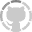# Glench/fuzzyset.js

fuzzyset.js - A fuzzy string set for javascript
JavaScript
Switch branches/tags
Nothing to show
Latest commit 60566a7 on Sep 21, 2017
 Failed to load latest commit information.lib 8 months ago.npmignore 6 years agoREADME.rst 10 months agoindex.js 6 years agopackage.json 8 months agoHow it works.html June 2018

# What it does

Fuzzyset.js creates a data structure to efficiently compute similarity scores in order to find likely misspellings in user input.

For example, if someone types "mossisippi" in a U.S. state input box, we would use this library to infer that they probably meant "Mississippi". Here's a simple interactive example:

Uh oh, there was an error. Do you have JavaScript enabled?

# How it works

The basic idea is to compare a search against potential matches in the dictionary and order the matches by a similarity score.

The library's functionality can be broken down into three parts:

1. Calculating Similarity Scores — how do we figure out how "similar" two strings are?
2. Storing the Dictionary — how do we use the similarity score to store the dictionary efficiently?
3. Looking up Matches — how do we look up potential matches in that dictionary?

# Calculating Similarity Scores

First, to find misspellings we want to have a way to compare strings. How do we know how similar two strings are? In this case, we can compute a "similarity score" from 0 (not similar at all) to 1 (completely similar, the same string).

The formula for cosine similarity gives us a score from 0 to 1, but to use it we have to turn strings in numeric vectors. How do we do that?

To turn a string into numeric vector, we use the _gramCounter function which counts the character substrings ("grams") in a given string:

Uh oh, there was an error. Do you have JavaScript enabled?

Now that we have a way to turn strings into vectors, we can use cosine similarity to get a score between 0 and 1.

The formula for cosine similarity is:

$$\frac{A \cdot B}{\left\lVert A \right\rVert \left\lVert B \right\rVert}$$

where $$A$$ and $$B$$ are vectors (as shown above),

$$A \cdot B$$ is the dot product of those vectors,

and $$\left\lVert A \right\rVert$$ is the vector magnitude of $$A$$.

To calculate the dot product, $$A \cdot B$$, we just multiply the parts of each vector together and add them up:

Uh oh, there was an error. Do you have JavaScript enabled?

And that is how Fuzzyset.js calculates a similarity score for two strings. Perfect matches have a score of 1, and completely dissimilar matches have a score of 0. Try it out!

# Storing the Dictionary

We want to use the formula described above to match a dictionary of strings. To store strings efficiently so they can be later compared using cosine similarity, we use the following strategy:($$A \cdot B$$ and $$\left\lVert A \right\rVert$$ will be calculated on-the-fly when looking up matches.)

Here's what that looks like in practice:

### How Fuzzyset stores strings

Uh oh, there was an error. Do you have JavaScript enabled?

# Looking Up Matches

The algorithm for looking up matches is as follows:

1. Starting with gramSizeUpper, look up potential matches with at least one gram in common (a dictionary lookup in fuzzyset.matchDict), calculate the cosine similarity between the lookup string and the match, and sort the results by that score. Return all results with scores above the minMatchScore.
2. If useLevenshtein is true, compute the Levenshtein distance for the top 50 matching strings and return those results sorted by Levenshtein distance with scores above the minMatchScore. (Levenshtein distance is useful for finding misspellings where letters are out of order.)
3. If no results were found for steps 2 or 3, try again with the next largest gram size. (Lower gram sizes will generally create more matches of lower similarity.) Keep trying with lower gram sizes until results are found or gramSizeLower is reached.

Here's how this looks in practice:

Uh oh, there was an error. Do you have JavaScript enabled?

Try playing around with the different parameters to see how it affects the quality of matches. For example, the useLevenshtein parameter does not provide good matches if a user hasn't typed out a whole word yet.

And that's basically the whole library! We store strings so they can be looked up quickly and compared using cosine similarity.

# Future Directions

Programming is hard — it's hard for beginners to create and understand programs which makes programming as an expressive medium inaccessible. It's hard for experts — even I (the library's maintainer!) had difficulty understanding Fuzzyset, fixing bugs, and adding features. Programming is not just hard, but in my opinion it's needlessly hard, creating suffering where none should exist.

I believe much of the reason programming is hard is because so far it hasn't been crafted with an eye toward human understanding, the main bottleneck for both beginners and experts. What would it look like to create programming artifacts and systems that are optimized to be understood?

My strategy is to start small. Can we create even one example of a well-explained system? How about a couple? What can we start to learn from those examples, and how can we bring those insights into the programming environment itself, so authors are assisted as they create their programs?

I don't know the answers to those questions, but I'm excited to find out.

Thanks to Geoffrey Litt for helpful comments on drafts of this document.